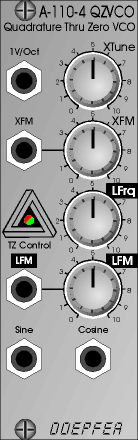﻿ A-110.4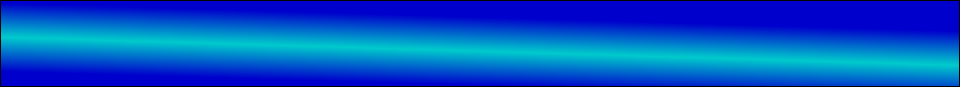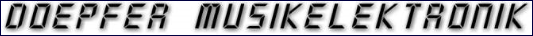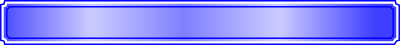Analogue  Modular  System  A-100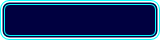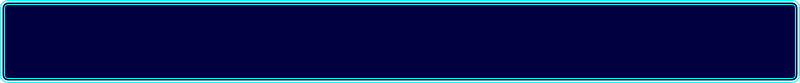WidthHPDepth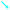mmCurrentmA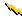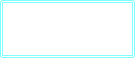Owners ManualNo manual available

8

60

10HP

tba

10HP

The A-110.4 is a Thru Zero Quadrature VCO. The term "quadrature" in this context, means that the oscillator outputs sine and cosine waveforms simultaneously. The term "Thru-Zero" means that  "negative" frequencies are generated, although  this a bit a misleading term as negative frequencies do not really exist. "Negative" means in this context that the sine/cosine waves will stop when the linear control voltage reaches 0V and continue with the opposite direction as the CV becomes negative.

The A-110.4 has two control sections: linear and exponential. The exponential section consists of the XTune control, the 1V/Oct input and the XFM input with the corresponding attenuator XFM. The exponential control voltage is the sum of these three voltages. The linear section consists of the LTune control and the LFM input with the corresponding attenuator LFM. The linear control voltage is the sum of these two voltages. A dual colour LED is used to display the polarity of the linear control voltage (green = positive, red = negative). The resolution pitch of the sine/cosine outputs is determined by the resulting control voltages of both sections. The linear section is used to control the pitch in a linear manner. When the LTune control (Linear Tune) is fully CW the module works like a normal Quadrature VCO (e.g. like the A-143-9) and the LED lights green. The pitch is then controlled by the exponential section with the manual Tune control and the exponential frequency control inputs 1V/Oct and XFM. 1V/Oct is used to control the pitch by a 1V/Oct CV source (e.g. key CV). XFM is used to apply an exponential frequency modulation with adjustable depth (e.g. from an LFO ). As the LTune control is turned counterclockwise starting from the fully CW position the frequency is lowered in a linear manner until the sine/cosine waves stop near the centre position of LTune (provided that no LFM signal is present). As the LTune control is moved from the centre towards the CCW position the waves start again but into reverse direction and the LED turns red. When the fully CCW position of LTune is reached the module works again like a normal Quadrature VCO. But much more exciting is the usage of the LFM input to modify the linear control voltage by an external control voltage (typically another VCO). Linear modulation by another oscillator using the thru zero feature generates audio spectra than cannot be obtained from an oscillator without the thru zero function. The reason is that a "normal" VCO will simply stop as the linear control voltage becomes zero or negative. But a thru zero VCO will start again with "negative" frequencies as the the linear control voltage becomes negative.

The main advantage of the A-110-4 compared to other Thru Zero VCOs is that the design used a sine/cosine core. The sine/cosine waves are not derived from other waveforms (e.g. sawtooth or triangle) by means of waveshaping. Rather the sine and cosine waves are the core of the VCO which results in very pure waves with a minimum of distortion and overtones.

Quadrature Thru Zero  Voltage Controlled Oscillator

A-110.4